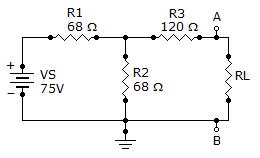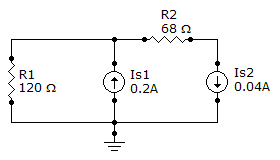# Electrical Engineering - Circuit Theorems and Conversions

Exercise : Circuit Theorems and Conversions - General Questions
6.
Determine IN for the circuit consisting of VS, R1, R2, and R3 shown in the given circuit.676 mA
245 mA
431 mA
75 mA
Explanation:
No answer description is available. Let's discuss.

7.
A 120 V voltage source has a source resistance, RS, of 60. The equivalent current source is
2 A
4 A
200 mA
400 mA
Explanation:
No answer description is available. Let's discuss.

8.
A 12 V source has an internal resistance of 90. If a load resistance of 20is connected to the voltage source, the load power, PL, is
2.38 mW
2.38 W
238 mW
23.8 W
Explanation:
No answer description is available. Let's discuss.

9.
In a two-source circuit, one source acting alone produces 12 mA through a given branch. The other source acting alone produces 10 mA in the opposite direction through the same branch. The actual current through the branch is
22 mA
12 mA
10 mA
2 mA
Explanation:
No answer description is available. Let's discuss.

10.
Find the current through R1 in the given circuit.0.16 A
0.24 A
0.2 A
0.04 A